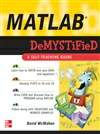# MATLAB Demystified: A Self-Teaching Guide

Need to Learn MATLAB? Problem SOLVED!

Get started using MATLAB right away with help from this hands-on guide. MATLAB Demystified offers an effective and enlightening method for learning how to get the most out this powerful computational mathematics tool.

Using an easy-to-follow format, this book explains the basics of MATLAB up front. You'll find out how to plot functions, solve algebraic equations, and compute integrals. You'll also learn how to solve differential equations, generate numerical solutions of ODEs, and work with special functions. Packed with hundreds of sample equations and explained solutions, and featuring end-of-chapter quizzes and a final exam, this book will teach you MATLAB essentials in no time at all.

• This self-teaching guide offers:
• The quickest way to get up and running on MATLAB
• Hundreds of worked examples with solutions
• Coverage of MATLAB 7
• A quiz at the end of each chapter to reinforce learning and pinpoint weaknesses
• A final exam at the end of the book
• A time-saving approach to performing better on homework or on the job

Simple enough for a beginner, but challenging enough for an advanced user, MATLAB Demystified is your shortcut to computational precision.

# 下载地址

• 本书版权归原作者所有，本站暂无下载，请购买正版！
•